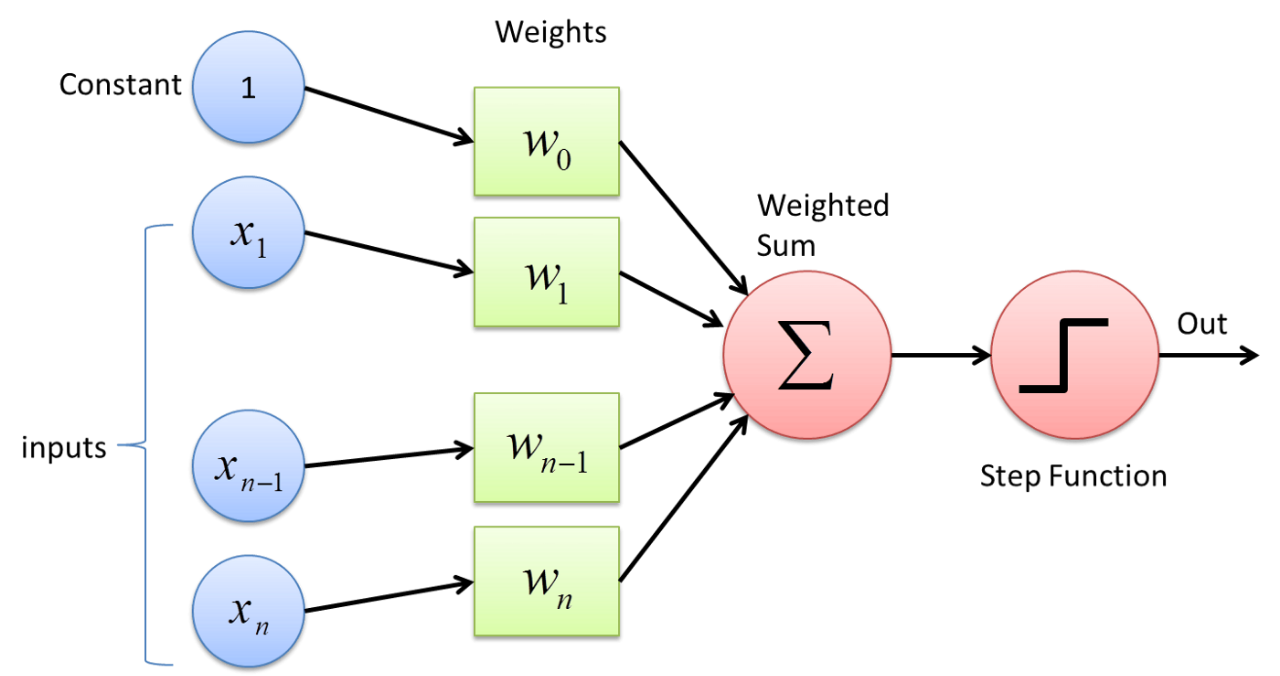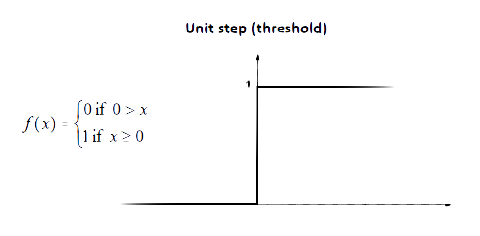# What the heck is a perceptron

A quick article about what a perceptron is, how it works, and why it is important.

In short, the perceptron is an algorithm. A very simple one at that. The algorithm it performs, in machine learning lingo, is known as binary classification.

Binary classification is the task of classifying elements into two groups. Hence the word binary — meaning 2.

All the algorithm does, it take an input and produce an output. The method by which the perceptron produces an output is called the weighted sum. It takes some input X, multiplies it by some weight W, adds in a bias B and then runs that value through some step function which classifies the weighted sum as a 0 or 1 (most of the time).

If there are multiple inputs, then there is a single weight assigned to each input and all of the inputs are multiplied by their corresponding weight and then summed together and added to the bias before being passed through the step function.

Here is visual of what a simple perceptron with n inputs may look like:This image depicts the inputs (represented as subscripts of X) each being multiplied by their corresponding weights, summed together, and passed through some step function that takes the weighted sum and produces either a 0 or a 1. Notice that there is a constant that is fed into the perceptron as well as the other inputs. This constant will always be 1 and when multiplied with the first weight, represents the bias. So, essentially, that weight is the bias — it is added to the sum of the weights without being multiplied first with an input.

A simple step function is depicted below:This step function takes some value. If the value is greater than or equal to 0, then it outputs 1, otherwise, it outputs 0.

## How is it useful at classifying things?

The perceptron is a subset of machine learning algorithms known as supervised learning. This means that when you teach a perceptron to learn to classify inputs into 2 categories, you give it the inputs and the labels that you want the perceptron to produce in the end (0 or 1 for binary classification, usually).

The perceptron initializes its weights randomly and get’s fed the inputs. It takes its final output from the step function and compares it to the label that you gave it for the specified set of inputs.

The perceptron can then see whether it was wrong at all and how wrong. It can quantify how wrong it is by producing a loss function which takes the perceptron’s output and the target label provided by you and produces a number. In essence, the loss function is saying, “this perceptron is 2.76 wrong” or “this perceptron is 8.92 wrong”, etc. Here is a common loss function for binary classification known as Squared Error:

!!Loss=(predicted-target)^2!!

So, in a simple example, if the perceptron was fed inputs and produced a 1, but the label assigned to the inputs was 0, then the loss of the perceptron would be:

!!Loss=(1-0)^2=1!!

The perceptron in this case was “1 wrong”.

It can then use this value to adjust the weights is various ways, one of which is known as Gradient Descent. In GD, you adjust the weights by subtracting the partial derivative of the loss function with respect to each weight. To actually figure out the derivatives, you would use the chain rule because the loss function is in actuality a composition of the weighted sum plus the bias, piped through the step function:

!!\frac{\partial Loss}{\partial w_i}=\frac{\partial Loss}{\partial y}\frac{\partial y}{\partial w_i}!!

If you are familiar with Calculus and derivatives, this concept shouldn’t be too difficult to understand and I am planning on writing an article on this soon. But it is beyond the scope of this high-level paper.

## What is a Binary Classifier?

This means that the input entered is mapped to 2 different categories.

Another way to describe this is by saying that the information entered into a perceptron is linearly separable:This means that the input entered can be separated by a single line.

## A perceptron is just like a linear function

One thing to note about a perceptron is that it is functions kind of line a linear function. For example, here is a function in slope-intercept form:

!!y=mx+b!!

When comparing this to a perceptron you can see they are much alike. The X is the input, the m is the weight, and the b is the bias. Here is what the perceptron value before it is run through the step function would look like:

!!y=\sum_{i=0}^{n} X_{i} W_{i}+B!!

Looks pretty similar, huh?

This high level overview of a perceptron is a good prep for learning about Neural Networks which are just perceptrons stacked on top of each other and gathered into layers.

Just keep learning!